SLVSB02B November   2013  – July 2014

PRODUCTION DATA.

1. Features
2. Applications
3. Description
4. Revision History
5. Device Comparison Table
6. Specifications
7. Detailed Description
1. 7.1 Overview
2. 7.2 Functional Block Diagram
3. 7.3 Feature Description
4. 7.4 Device Functional Modes
8. Application and Implementation
1. 8.1 Application Information
2. 8.2 Typical Application
1. 8.2.1 Design Requirements
2. 8.2.2 Application Curves
3. 8.3 System Example
9. Power Supply Recommendations
10. 10Layout
11. 11Device and Documentation Support
1. 11.1 Device Support
2. 11.2 Documentation Support
5. 11.5 Electrostatic Discharge Caution
6. 11.6 Glossary
12. 12Mechanical, Packaging, and Orderable Information

• DSS|12
• DSS|12

## 8 Application and Implementation

### 8.1 Application Information

The TPS6274x devices are a step down converter family featuring typ. 360nA quiescent current and operating with a tiny 2.2µH inductor and 10µF output capacitor. This new DCS-ControlTM based devices extend the light load efficiency range below 10µA load currents. TPS62740 supports output currents up to 300mA, TPS62742 up to 400mA. The devices operate from rechargeable Li-Ion batteries, Li-primary battery chemistries such as Li-SOCl2, Li-MnO2 and two or three cell alkaline batteries.

### 8.2 Typical Application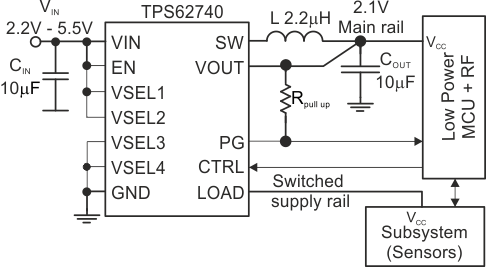Figure 7. TPS62740 Typical Application CircuitFigure 8. TPS62742 Typical Application Circuit

#### 8.2.1 Design Requirements

The TPS6274x is a highly integrated DC/DC converter. The output voltage is set via a VSEL pin interface without any additional external components. For proper operation only a input- and output capacitor and an inductor is required. The integrated load switch doesn't require a capacitor on its LOAD pin. Table 2 shows the components used for the application characteristic curves.

### Table 2. Components for Application Characteristic Curves

Reference Description Value Manufacturer
TPS62740/42 360nA Iq step down converter Texas Instruments
CIN, COUT, CLOAD Ceramic capacitor GRM188R60J106M 10µF Murata
L Inductor LPS3314 2.2µH Coilcraft

#### Detailed Design Procedure

Table 3 shows the recommended output filter components. The TPS6274x is optimized for operation with a 2.2µH inductor and with 10µF output capacitor.

### Table 3. Recommended LC Output Filter Combinations

Inductor Value [µH](2) Output Capacitor Value [µF](1)
4.7µF 10µF 22µF
2.2 (3)
(1) Capacitance tolerance and bias voltage de-rating is anticipated. The effective capacitance can vary by 20% and -50%.
(2) Inductor tolerance and current de-rating is anticipated. The effective inductance can vary by 20% and -30%.
(3) This LC combination is the standard value and recommended for most applications.

#### 8.2.1.1 Inductor Selection

The inductor value affects its peak-to-peak ripple current, the PWM-to-PFM transition point, the output voltage ripple and the efficiency. The selected inductor has to be rated for its DC resistance and saturation current. The inductor ripple current (ΔIL) decreases with higher inductance and increases with higher VIN or VOUT and can be estimated according to Equation 1.

Equation 2 calculates the maximum inductor current under static load conditions. The saturation current of the inductor should be rated higher than the maximum inductor current, as calculated with Equation 2. This is recommended because during a heavy load transient the inductor current rises above the calculated value. A more conservative way is to select the inductor saturation current above the high-side MOSFET switch current limit, ILIMF.

Equation 1.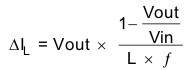Equation 2.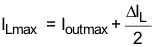With:

f = Switching Frequency
L = Inductor Value
ΔIL= Peak to Peak inductor ripple current
ILmax = Maximum Inductor current

In DC/DC converter applications, the efficiency is essentially affected by the inductor AC resistance (i.e. quality factor) and by the inductor DCR value. Increasing the inductor value produces lower RMS currents, but degrades transient response. For a given physical inductor size, increased inductance usually results in an inductor with lower saturation current.

The total losses of the coil consist of both the losses in the DC resistance (RDC) and the following frequency-dependent components:

• The losses in the core material (magnetic hysteresis loss, especially at high switching frequencies)
• Additional losses in the conductor from the skin effect (current displacement at high frequencies)
• Magnetic field losses of the neighboring windings (proximity effect)

The following inductor series from different suppliers have been used:

### Table 4. List Of Inductors(1)

INDUCTANCE [µH] DIMENSIONS [mm3] INDUCTOR TYPE SUPPLIER
2.2 3.3 x 3.3 x 1.4 LPS3314 Coilcraft
2.2 2.5 x 3.0 x 1.5 VLF302515MT TDK
2.2 2.0 × 1.2 × 1.0 MIPSZ2012 2R2 FDK
2.2 2.5 x 2.0 x 1.2 MIPSA2520 2R2 FDK
2.2 2.0 x 1.2 x 1.0 MDT2012CH2R2 TOKO

#### 8.2.1.2 DC/DC Output Capacitor Selection

The DCS-Control™ scheme of the TPS6274x allows the use of tiny ceramic capacitors. Ceramic capacitors with low ESR values have the lowest output voltage ripple and are recommended. The output capacitor requires either an X7R or X5R dielectric. Y5V and Z5U dielectric capacitors, aside from their wide variation in capacitance over temperature, become resistive at high frequencies. At light load currents, the converter operates in Power Save Mode and the output voltage ripple is dependent on the output capacitor value and the PFM peak inductor current. A larger output capacitors can be used, but it should be considered that larger output capacitors lead to an increased leakage current in the capacitor and may reduce overall conversion efficiency. Furthermore, larger output capacitors impact the start up behavior of the DC/DC converter.

#### 8.2.1.3 Input Capacitor Selection

Because the buck converter has a pulsating input current, a low ESR input capacitor is required for best input voltage filtering to ensure proper function of the device and to minimize input voltage spikes. For most applications a 10µF is sufficient. The input capacitor can be increased without any limit for better input voltage filtering.

Table 5 shows a list of tested input/output capacitors.

### Table 5. List Of Capacitors(1)

CAPACITANCE [μF] SIZE CAPACITOR TYPE SUPPLIER
10 0603 GRM188R60J106ME84 Murata

#### 8.2.2 Application Curves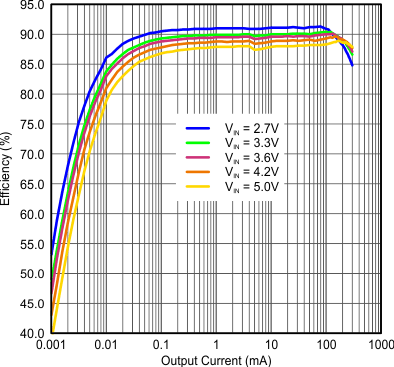COUT = 10 µF (0603) CTRL = GND L = 2.2 µH (LPS3314 2R2)
Figure 9. Efficiency VOUT = 1.8VCOUT = 10 µF, CTRL = GND. L = 2.2 µH (VLF302515)
Figure 11. Efficiency VOUT = 3.3V TPS62742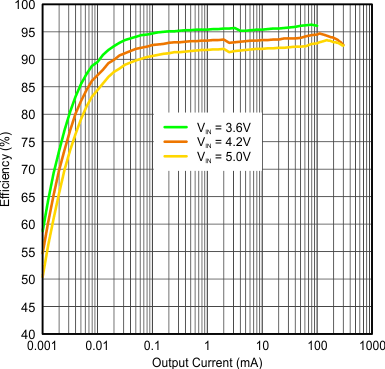COUT = 10 µF (0603) CTRL = GND L = 2.2 µH (LPS3314 2R2)
Figure 13. Efficiency VOUT = 3.3V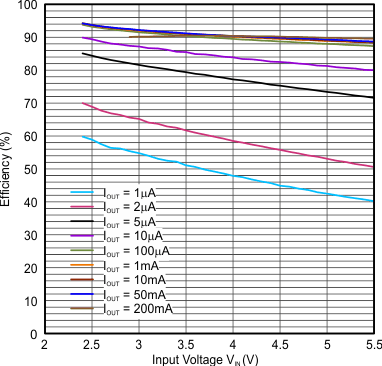COUT = 10 µF (0603) CTRL = GND L = 2.2 µH (LPS3314 2R2)
Figure 15. Efficiency VOUT = 2.1V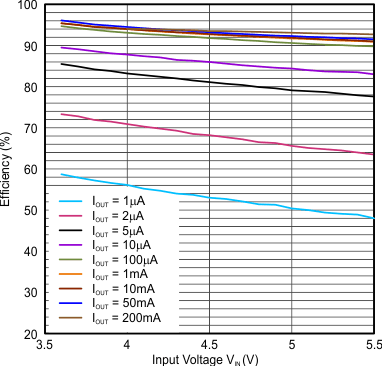COUT = 10 µF (0603) CTRL = GND L = 2.2 µH (LPS3314 2R2)
Figure 17. Efficiency VOUT = 3.3V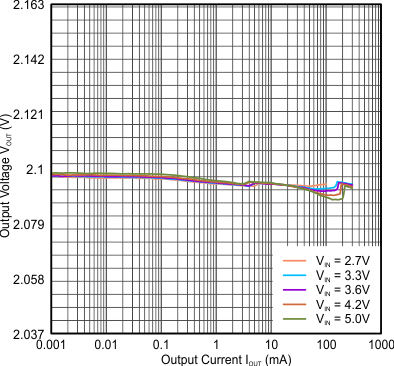COUT = 10 µF (0603) CTRL = GND L = 2.2 µH (LPS3314 2R2)
Figure 19. Output Voltage VOUT = 2.1V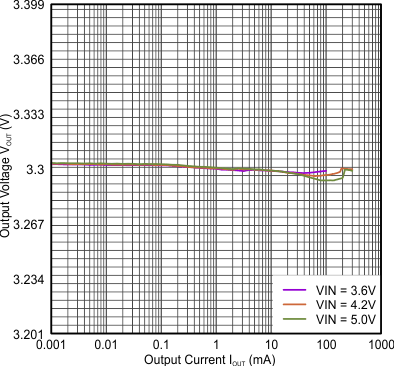COUT = 10 µF (0603) CTRL = GND L = 2.2 µH (LPS3314 2R2)
Figure 21. Output Voltage VOUT = 3.3V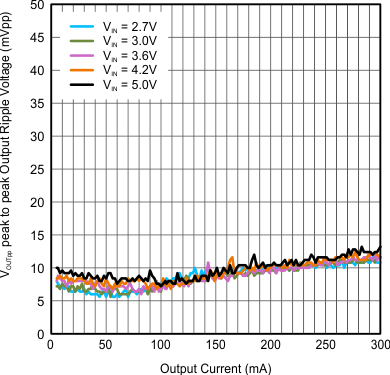COUT = 10 µF (0603) CTRL = GND L = 2.2 µH
Figure 23. Typical Output Ripple Voltage VOUT = 1.8V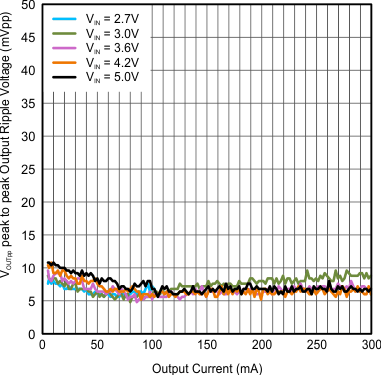COUT = 10 µF (0603) CTRL = GND L = 2.2 µH
Figure 25. Typical Output Ripple Voltage VOUT = 2.1V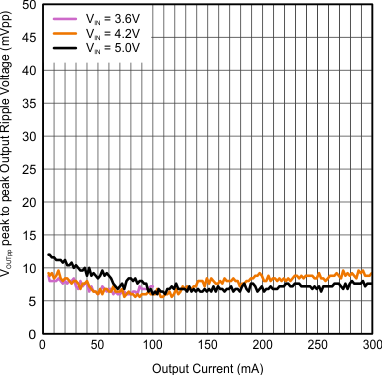COUT = 10 µF (0603) CTRL = GND L = 2.2 µH
Figure 27. Typical Output Ripple Voltage VOUT = 3.0V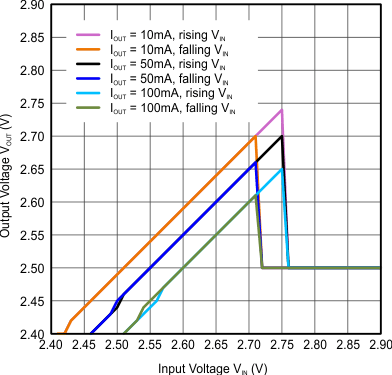L = 2.2 µH (LPS3314)
Figure 29. 100% Mode Transition VOUT 2.5V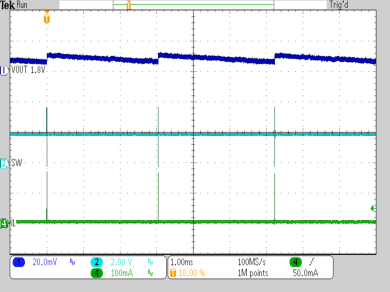VIN = 3.6 V IOUT = 10 µA L = 2.2 µH COUT = 10 µF CTRL = GND
Figure 31. Typical Operation ILoad = 10µA VOUT = 1.8V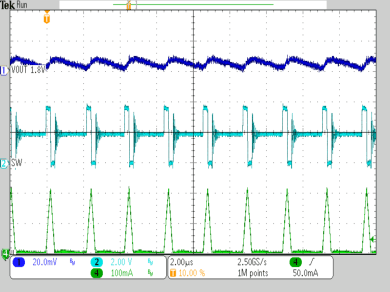VIN = 3.6 V IOUT = 25 mA L = 2.2 µH COUT = 10 µF CTRL = GND
Figure 32. Typical Operation ILoad = 25mA, VOUT = 1.8VVIN = 3.6 V IOUT = 50 µA to 10 mA L = 2.2 µH COUT = 10 µF CTRL = GND
Figure 34. Load Transient Response VOUT = 1.8V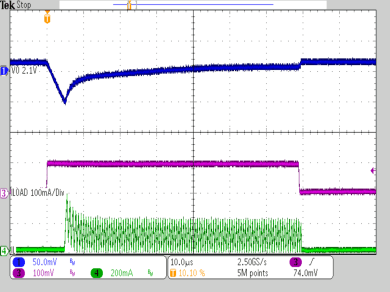VIN = 3.6 V, VOUT = 2.1 V L = 2.2 µH COUT = 10 µF Loadstep at VOUT 0 mA to 100 mA, 1 µs rise/ fall time, 70 µs / 7 ms
Load Transient Response CTRL = GNDVIN = 3.6 V / 4.2 V VOUT = 2.1 V L = 2.2 µH COUT = 10 µF CTRL = GND
Figure 37. Line Transient Response IOUT=10mAVIN = 3.6 V IOUT = 50 µA to 300 mA L = 2.2 µH COUT = 10 µF CTRL = GND
Figure 39. AC Load Sweep VOUT = 2.1V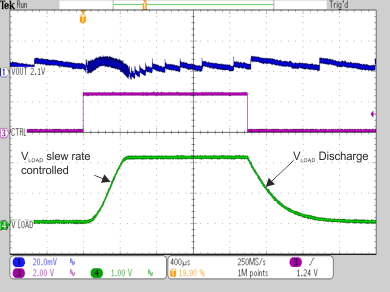VIN = 3.6 V VOUT = 2.1 V ILOAD = 0 mA IOUT = 0 mA COUT = 10 µF CLOAD = 10 µF L = 2.2 µH
Figure 41. Load Output On / OffVIN = 3.6 V VOUT = 2.1 V CTRL = GND ROUT = 100 Ω COUT = 10 µF L = 2.2 µH
VOUT Ramp Up After EnableVIN = 3.6 V Ramp up / Down COUT = 10 µF CTRL = GND VSEL 3+4 toggled IOUT = 5 mA L = 2.2 µH VSEL 1+2 = GND
Dynamic Output Voltage Scaling
VOUT = 1.8V/3.0V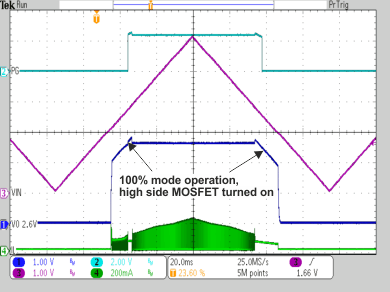COUT = 10 µF L = 2.2 µH CTRL = GND VIN = ramp up/down 0 V to 5 V, 150 ms, Output resistance 50 Ω
Input Voltage Ramp Up/Down VOUT = 2.6V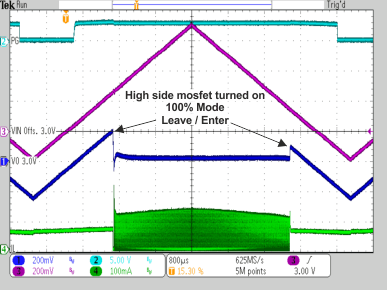VOUT = 3.0 V COUT = 10 µF L = 2.2 µH, CTRL = GND VIN = ramp up /down 2.8 V to 3.7 V, Output resistance 50 Ω
Enter/Leave 100% Mode Operation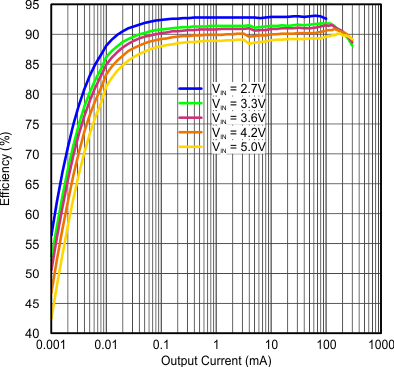COUT = 10 µF (0603) CTRL = GND L = 2.2 μH (LPS3314 2R2)
Figure 10. Efficiency VOUT = 2.1V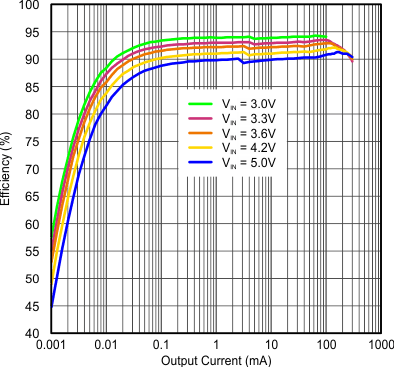COUT = 10 µF (0603) CTRL = GND L = 2.2 µH (LPS3314 2R2)
Figure 12. Efficiency VOUT = 2.5V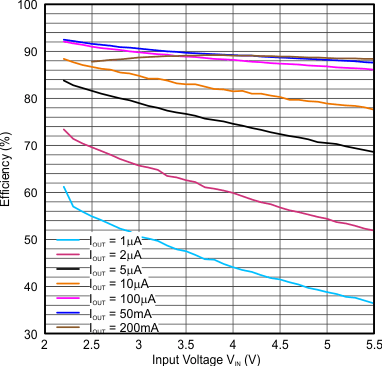COUT = 10 µF (0603) CTRL = GND L = 2.2 µH (LPS3314 2R2)
Figure 14. Efficiency VOUT = 1.8V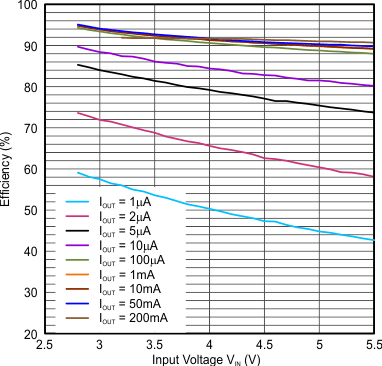COUT = 10 µF (0603) CTRL = GND L = 2.2 µH (LPS3314 2R2)
Figure 16. Efficiency VOUT = 2.5V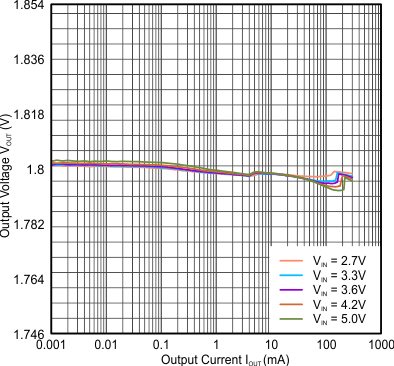COUT = 10 µF (0603) CTRL = GND L = 2.2 µH (LPS3314 2R2)
Figure 18. Output Voltage VOUT = 1.8V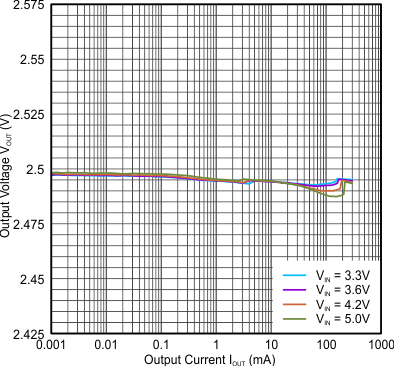COUT = 10 µF (0603) CTRL = GND L = 2.2 µH (LPS3314 2R2)
Figure 20. Output Voltage VOUT = 2.5V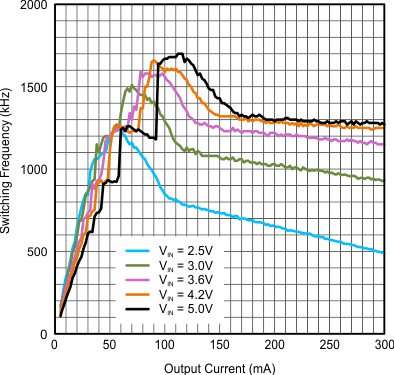COUT = 10 µF L = 2.2 µH
Figure 22. Typical Switching Frequency VOUT = 1.8V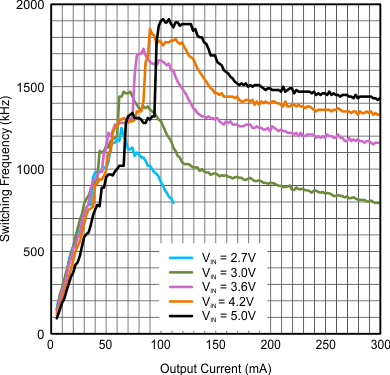COUT = 10 µF L = 2.2 µH
Figure 24. Typical Switching Frequency VOUT = 2.1V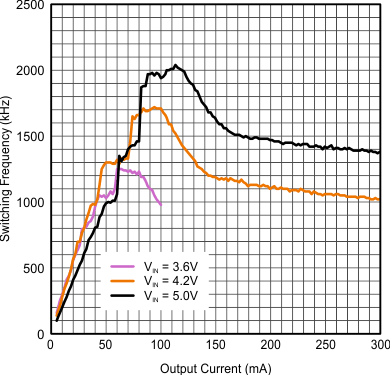COUT = 10 µF L = 2.2 µH
Figure 26. Typical Switching Frequency VOUT = 3.0V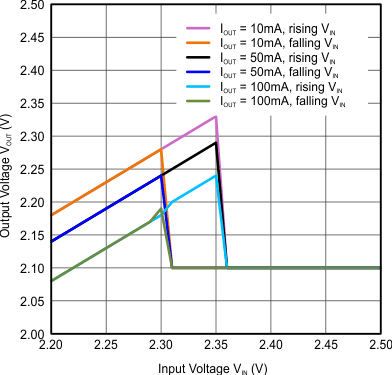L = 2.2 µH (LPS3314)
Figure 28. 100% Mode Transition VOUT 2.1V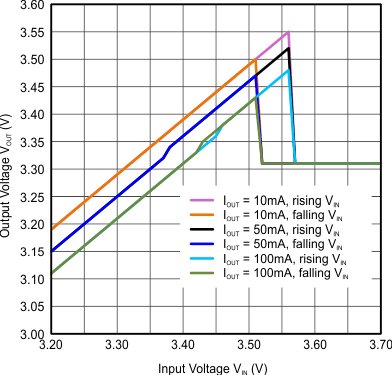L = 2.2 µH (LPS3314)
Figure 30. 100% Mode Transition VOUT 3.3V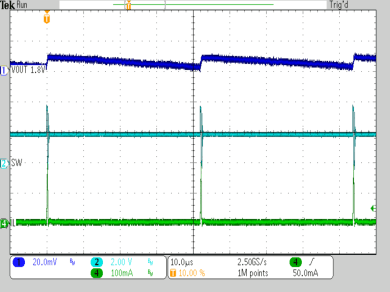VIN = 3.6 V IOUT = 1 mA L = 2.2 µH COUT = 10 µF CTRL = GND
Typical Operation ILoad = 1ma, VOUT = 1.8V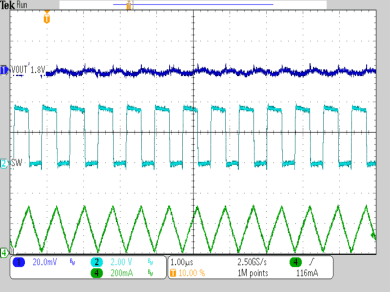VIN = 3.6 V IOUT = 150 mA L = 2.2 µH COUT = 10 µF CTRL = GND
Figure 33. Typical Operation ILoad = 150ma, VOUT = 1.8VVIN = 3.6 V IOUT = 0.5 mA to 150 mA L = 2.2 µH COUT = 10 µF CTRL = VIN
Figure 35. Load Transient Response VOUT = 2.1V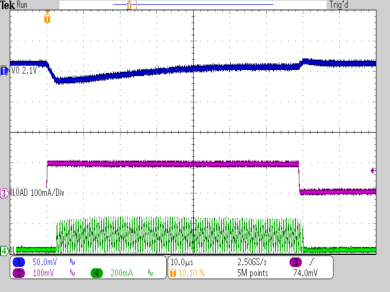VIN = 3.6 V, VOUT = 2.1 V L = 2.2 µH, COUT = 10 µF Loadstep at VOUT 0 mA to 100 mA, 1 µs rise/fall time; 70 µs / 7 ms
Figure 36. Load Transient Response CTRL = VINVIN = 3.6 V / 4.2 V VOUT = 2.1 V L = 2.2 µH COUT = 10 µF CTRL = GND
Figure 38. Line Transient Response IOUT = 100mAVIN = 3.6 V, VOUT = VLOAD= 2.1 V CTRL = VIN IOUT = 0 mA CLOAD = 10 µF L = 2.2 µH COUT = 10 µF ILOAD = 0 to 50 mA to 0 mA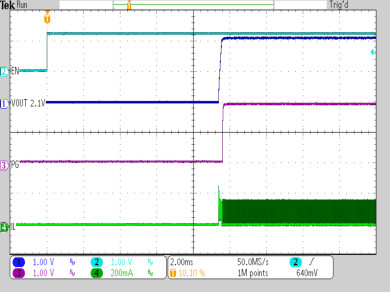VIN = 3.6 V VOUT = 2.1 V CTRL = GND ROUT = 100 Ω COUT = 10 µF L = 2.2 µH
Device Enable And Start UpVIN = 3.6 V VOUT = VLOAD = 2.1 V CTRL = VIN ROUT = 100 Ω, COUT = CLOAD = 10 µF L = 2.2 µH ILOAD = 0 mA
VOUT Ramp Up With Activated Load Switch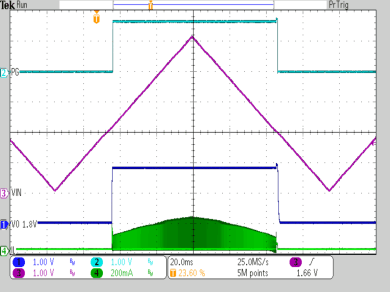COUT = 10 µF L = 2.2 µH CTRL = GND VIN = ramp up/down 0 V to 5 V, 150 ms, Output resistance 50 Ω
Input Voltage Ramp Up/Down
VOUT = 1.8V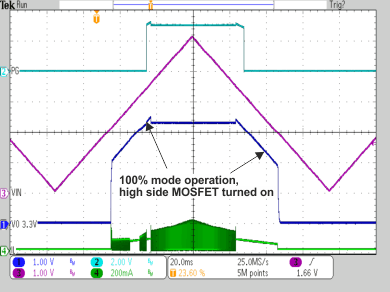COUT = 10 µF L = 2.2 µH CTRL = GND VIN = ramp up/down 0 V to 5 V, 150 ms, Output resistance 50 Ω
Input Voltage Ramp Up/Down VOUT = 3.3V

### 8.3 System Example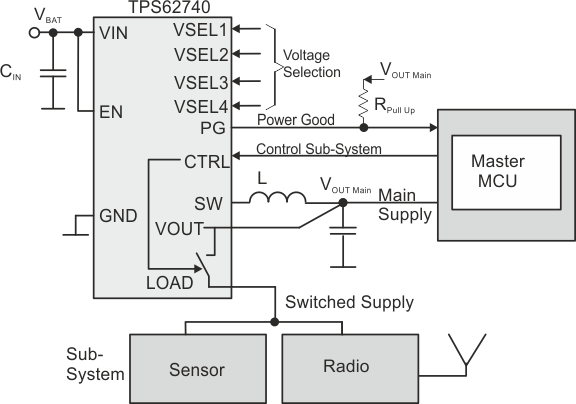Figure 42. Example Of Implementation In A Master MCU Based System